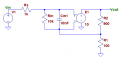# Input impedance vs imput capacitance

#### anhnha

Joined Apr 19, 2012
901
If a circuit has high input impedance, does it mean that the circuit also has high input capacitance?
Is there a correlation between them?

#### #12

Joined Nov 30, 2010
18,224

#### anhnha

Joined Apr 19, 2012
901

#### panic mode

Joined Oct 10, 2011
2,451
you need to consider that circuit as three stages: signal source (producing Vin), interconnect (medium, aka transmission line), load (some VLSI device that reads Vout as input signal).
load is not represented as a component (impedance). but if we have all things same (interconnect) and ONLY change impedance at Vout, then resitance R of interconnect does not matter (much smaller than your huge Z=Rout which is near infinity) but capacitance of the interconnect itself becomes significant factor.

•anhnha

#### anhnha

Joined Apr 19, 2012
901
Thanks, that makes sense. Actually, I don't understand the part "high input impedance of the receiver gives rise to high input capacitance".
I just made a draft calculation. It seems that input capacitance doesn't change.
http://i.imgur.com/FKbWVKd.jpg

Rint: interconnect resistance
Rout: resistance of output network

#### Papabravo

Joined Feb 24, 2006
20,155
If a circuit has high input impedance, does it mean that the circuit also has high input capacitance?
Is there a correlation between them?
Check the units. Capacitance is a real number with units of Farads. Impedance is a complex number whose magnitude has units of Ohms and whose phase is expressed in degrees or radians. How could they be correlated? One is frequency dependent and one is not, again how could the be correlated?

#### anhnha

Joined Apr 19, 2012
901
Actually, I didn't intend to mean correlation that way (maybe that is because of my English). I think a better work is "result in". I was confused if a high input impedance results in high input capacitance.

#### #12

Joined Nov 30, 2010
18,224
I don't understand the part "high input impedance of the receiver gives rise to high input capacitance".
I think the instruction page is wrong, and that would explain why it is difficult to understand.
I have designed high input impedance circuits with only picofarads of capacitance (because low capacitance was an important part of making the circuit work properly). The fact that high input impedance, "gives rise to" high input capacitance is not (a fact).

•anhnha

#### sailorjoe

Joined Jun 4, 2013
364
That's right, the instructions are imprecise. What they're trying to say is that, no matter what you do, there is always at least a little bit of capacitance at the input to a signal receiver, the part with ideally infinite impedance. In the circuit, R would be infinite. But since C is always there, the large R increases the time constant of R times C. So the high input impedance doesn't really "create" the high capacitance, but it magnifies the effect of the capacitance.

•anhnha

#### Jony130

Joined Feb 17, 2009
5,445
Try exam this non-inverter amplifier with open loop gain Aol = 10V/V; and Aol = 100V/V;
And Cin = 10nF ; Rin = 10kΩ and Rs = 1kΩ;For Aol = 1V/V the -3dB is at F ≈ 0.16/RsCin ≈ 16kHz and Rin ≈ 10kΩ
But as Aol increasing the Rin also increases and -3dB drops as if Cin was reduced.
For example for Aol = 10V/V -3dB is at F ≈ 0.16/(Rs*Cin/2) ≈ 32kHz and Rin ≈ 2*Rin = 20kΩ
This "2" is a (1 + Aol * R1/(R1+R2))
And as Aol increase to infinity (ideal opamp) Rin and -3dB rise to infinity.

•anhnha